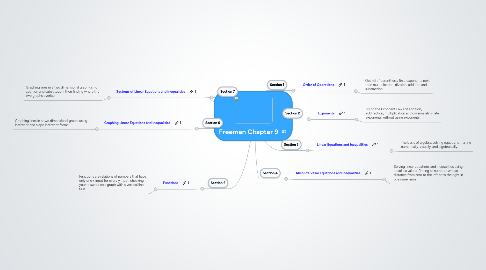# Freeman Chapter 9

Get Started. It's FreeFreeman Chapter 9## 1. Section 5

### 1.1. Functions

1.1.1. Functions are relations of numbers that have only one x input for every y input, checking your answers on a graph with a vertical line test.

## 2. Section 6

### 2.1. Graphing Linear Equations and Inequalities

2.1.1. Graphing lines in a two dimensional graph, using intercept and slope intercept forms.

## 3. Section 7

### 3.1. Systems of Linear Equations and Inequalities

3.1.1. Graphing lines in a two dimensional graph using addition and substitution, then finding where the two graphs overlap.

## 4. Section 2

### 4.1. Exponents

4.1.1. Using the Exponent Laws of addition, subtraction, multiplication and division, eliminate exponents without using exponents.

## 5. Section 3

### 5.1. Linear Equations and Inequalities

5.1.1. The basis of algebra, solving equations in a line numerically, visually, and algebraically.

## 6. Section 4

### 6.1. Absolute Value Equations and Inequalities

6.1.1. Solving linear equations and inequalities using absolute values, finding all numbers whose distance from zero to the left or to the right in one dimension.

## 7. Section 1

### 7.1. Order of Operations

7.1.1. Get rid of parenthesis first, exponents next, then multiplication, division, addition and subtraction.